Vectors and conics

This free course is available to start right now. Review the full course description and key learning outcomes and create an account and enrol if you want a free statement of participation.

Free course

4.4 Parabola (e = 1)

A parabola is defined to be the set of points P in the plane whose distances from a fixed point F are equal to their distances from a fixed line d. We obtain a parabola in standard form if

1. the focus F lies on the x-axis, and has coordinates (a, 0), where a > 0;

2. the directrix d is the line with equation x = −a.

Thus the origin lies on the parabola, since it is equidistant from F and d.

Let P (x, y) be an arbitrary point on the parabola, and let M be the foot of the perpendicular from P to the directrix. Since FP = PM, by the definition of the parabola, it follows that FP2 = PM2; that is,

(x − a)2 + (y − 0)2 = (x + a)2.

Multiplying out the brackets, we obtain x2 − 2ax + a2 + y2 = x2 + 2ax + a2, which simplifies to the equation y2 = 4ax.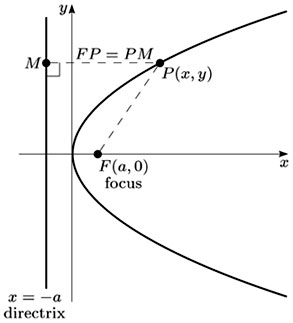Each point with coordinates of the form (at2, 2at), where t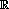, lies on the parabola, since (2at)2 = 4a × at2. Conversely, we can write the coordinates of each point on the parabola in the form (at2, 2at). If we choose t = y/(2a), then y = 2at and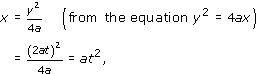as required. Thus there is a one-one correspondence between the real numbers t and the points of the parabola.

We call the x-axis the axis of a parabola in standard form, since the parabola is symmetric with respect to this line, and we call the origin the vertex of a parabola in standard form, since it is the point of intersection of the axis with the parabola. A parabola has no centre.

We summarise these facts as follows:

Parabola in standard form

A parabola in standard form has equation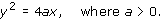It can also be described by the parametric equations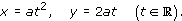It has focus (a, 0) and directrix x = −a; its axis is the x-axis and its vertex is the origin.

Example 8

Consider the parabola with equation y2 = 2x and parametric equations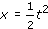, y = t (t).

• (a) Write down the focus, vertex, axis and directrix of the parabola.

• (b) Determine the equation of the chord that joins the two distinct points P and Q with parameters t1 and t2, respectively. Determine the condition on t1 and t2 such that the chord PQ passes through the focus of the parabola. Such a chord is called a focal chord.

• (a) The parabola is in standard form, where 4a = 2, that is,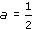. It follows that its focus is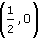, its vertex is (0, 0), its axis is the x-axis and the equation of its directrix is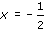.

• (b) The coordinates of P and Q are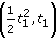and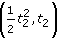, respectively.

•• We must consider the cases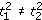and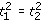separately.

• We suppose first that. Then the gradient of PQ is

•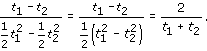• Sincelies on the line PQ, the equation of PQ is

•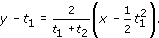• Multiplying both sides by t1 + t2, we obtain

•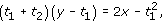• so

•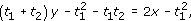• and hence

•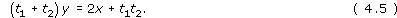• If, then t1 = −t2 (as t1t2, since P and Q are distinct). When t1 = −t2, PQ is parallel to the y-axis and the equation of PQ is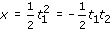• that is, 0 = 2x + t1t2.

• Thus equation (4.5) is the equation of PQ in the case t1 = −t2 also, for then t1 + t2 = 0.

Thus PQ passes through the focusif and only ifsatisfies equation (4.5), which is the case if and only if

• (t1 + t2)0 = 1 + t1t2;

• that is, if and only if t1t2 = −1.

Example 55

Consider the parabola with equation y2 = x and parametric equations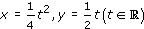.

• (a) Write down the focus, vertex, axis and directrix.

• (b) Determine the equation of the chord that joins the two distinct points P and Q with parameters t1 and t2, respectively.

• (c) Determine the condition on t1 and t2, and so on P and Q, such that the focus of the parabola is the midpoint of the chord PQ.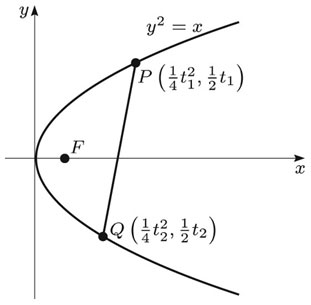• (a) The parabola is in standard form, where 4a = 1, that is,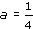. It follows that the focus is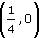, the vertex is (0, 0), the axis is the x-axis, and the equation of the directrix is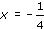.

• (b) The coordinates of P and Q are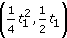and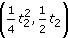, respectively. It follows that if, then the gradient of PQ is

•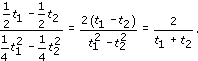• Since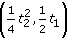lies on the line PQ, it follows that the equation of PQ is

•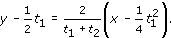• Multiplying both sides by 2(t1 + t2), we obtain

•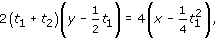• so

•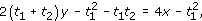• and hence

•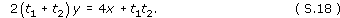• If, then t1 = −t2 (as t1 ≠ −t2, since P and Q are distinct). If t1 = −t2, then PQ is parallel to the y-axis and the equation of PQ is

•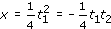• that is,

•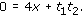• Thus equation (S.18) is the equation of PQ in the case t1 = −t2 also, for then t1 + t2 = 0.

• (c) The midpoint of PQ is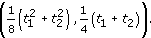• This is the focusif and only if

•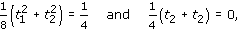• which is the case if and only if t2 = −t1 and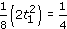, that is,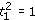and so t1 = ± 1. Thus the midpoint of PQ is the focus if and only if P and Q are the points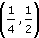and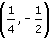.

• Remark: The focus is the midpoint of PQ if and only if PQ is the chord through the focus parallel to the y-axis.

M208_1